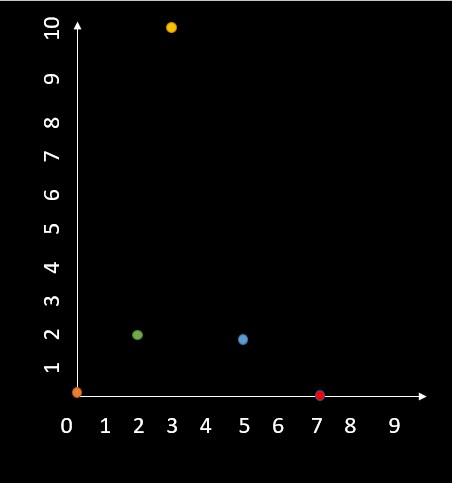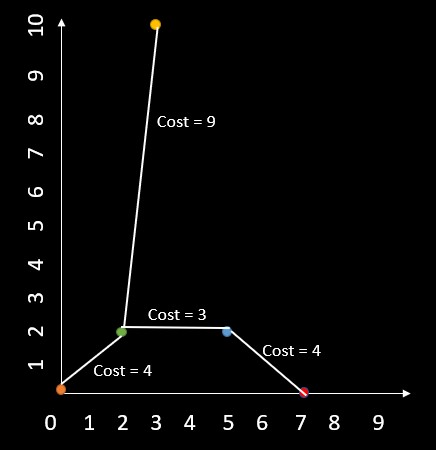×

Search anything:

# Minimum cost to connect all points (using MST)

#### Algorithms Minimum Spanning Tree (MST) Graph AlgorithmsIn this article at OpenGenus, we will understand how to solve the minimum cost to connect all the points problem using Minimum Spanning Tree.

Pre-requisites

### Problem statement

A vector named `points` is given, it has integer coordinates of some points in a 2D-plane, where `points[i] = [xi, yi]`. The distance between two points is calculated by manhattan distance between them as: `|xi - xj| + |yi - yj|`, where `|val|` denotes the absolute value of `val`. The cost to connect two points is equal to the distance between those two points.

Return the minimum cost to connect all the points. If there is exactly one simple path between two points then those two points are said to be connected.

``````Input: points = [[0,0],[2,2],[3,10],[5,2],[7,0]]
Output: 20
Explanation:
The below diagram shows how it's connected. Here you can see that there is a unique path between every pair of points.
``````### Observation

• Question is asking to find the minimum cost to make all the points connected in the 2D-plane.
• To find the distance use the manhattan distance formula.
• The points represent the `x` and `y` coordinate of each of the point.
• Cost is equal to the distance.

### Approach

Intuitive steps

• Initialize `n` as the number of points, `res` as the minimum cost to connect all points, `i` as the starting point, and connect as the number of connected points. Also, create a boolean vector `visited` to keep track of the points that have been added to the minimum spanning tree.
• Create a priority queue `pq` to store the edges and each edge is represented as a pair of (distance, index), and the priority of the edge is determined by its distance.
• While the number of connected points is less than the total number of points then we will do the necessary operations to find the cost.
• Return the cost after necessary calculations.

Explained

• As explained earlier, here we are given a 2D vector `points` as input, where each element `points[i]` represents the coordinates of the `i-th` point in the form of `[xi, yi]`.
• Before we start the program we will initialize some variables, `n` which is the number of points, `res` which would contain the minimum cost to all thee points, `i` the starting point and `connect` would be the number of connected points. We will also have a vector of boolean values `visited` to keep track of the points that have been added to the minimum spanning tree.
• Here we will use a priority queue `pq` to store the edges, where each edge is represented as a pair of `distance, index` where the priority of the edge is determined by its distance.
• The distance between two points is calculated using the Manhattan distance formula, which is given by the sum of the absolute differences of their `x` and `y` coordinates. The formula is: `Manhattan distance = |x1 - x2| + |y1 - y2|`.
• Since we got all the general points, lets go for the execution. First we will check if `connect < n` and we will increment the `connect` by `1` at the same time, this is done as we will be connecting one node. Now inside the loop we will make the `visited[i] = true` since we are visiting that point now. Now we will create a for loop to check if `j th` point is visited or not by an `if` statement `if(!visited[j])`, if its not visited we will push the distance between the `i th` point and `j th` point as the first element and the `j th` node as the second element of the node into the priority queue.
• Now after this loop we will take the first top element in the priority queue, which has not visited yet. We are checking if its visited or not again because even after checking whether a point is visited before adding its edges to the priority queue, there may be cases where a visited point is connected to an unvisited point by an edge that has already been added to the priority queue. In such cases, we need to remove the edge from the priority queue to ensure that we do not add it to the minimum spanning tree. We will do this using a while loop.
• After getting the required element, we will add the distance which would be equal to the cost to the `res` variable. Then we will make `i` the newly connected variable and then `pop()` the element.
• After the for loop we will return the `res` variable as the cost.
``````#include<bits/stdc++.h>
using namespace std;

int minCostConnectPoints(vector<vector<int>>& points) {
int n=points.size(), res=0, i=0, connect=0;
vector<bool>visited(n);
priority_queue<pair<int,int>, vector<pair<int,int>>, greater<pair<int,int>>>pq;
while(++connect < n){
visited[i]=true;
for(int j=0;j<n;j++){
if(!visited[j]){
pq.push({(abs(points[i]-points[j])+abs(points[i]-points[j])), j});
}
}
while(visited[pq.top().second])
pq.pop();
res+=pq.top().first;
i=pq.top().second;
pq.pop();
}
return res;
}

int main(){
int n;
cout<<"Enter number of points: ";
cin>>n;
vector<vector<int>>v(n);
cout<<"Enter x and y coordinates: ";
for(int i=0;i<n;i++){
int x,y;
cin>>x>>y;
v[i].push_back(x);
v[i].push_back(y);
}
cout<<"The minimum cost is: "<<minCostConnectPoints(v);
return 0;
}
``````

Output

``````Enter number of points: 5
Enter x and y coordinates: 0 0
2 2
3 10
5 2
7 0
The minimum cost is: 20
``````### Complexities

Here the time complexity of the above program will be `O(N^2 _ log N)`, where `N` is the number of points in the input array. Here we are building a minimum spanning tree using Prim's algorithm, which requires visiting every vertex at least once, resulting in `O(N)` operations. For each vertex, we also need to calculate the manhattan distance between it and every other unvisited vertices, which requires `O(N)` operations. This will result in `O(N^2)` time complexity. But we are also using a priority queue to keep track of the edges between the visited and unvisited vertices. In the worst case we can have up to `N(N-1)/2` edges, and inserting each edge takes `O(log N)` time. Hence the total time complexity is `O(N^2 _ log N)`.

The space complexity of the program will be `O(N)`, which is the space to store the visited array and the priority queue.

This makes it an efficient solution for small to medium-sized inputs, but it may be too slow for very large inputs.

With this article at OpenGenus, you must have the complete idea to solve this problem of Minimum cost to connect all the points using MST.

#### Aswin Shailajan

Aswin Shailajan is a B. Tech Computer Science student at Vellore Institute of Technology and is a SDE, Intern at OpenGenus.

Minimum cost to connect all points (using MST)